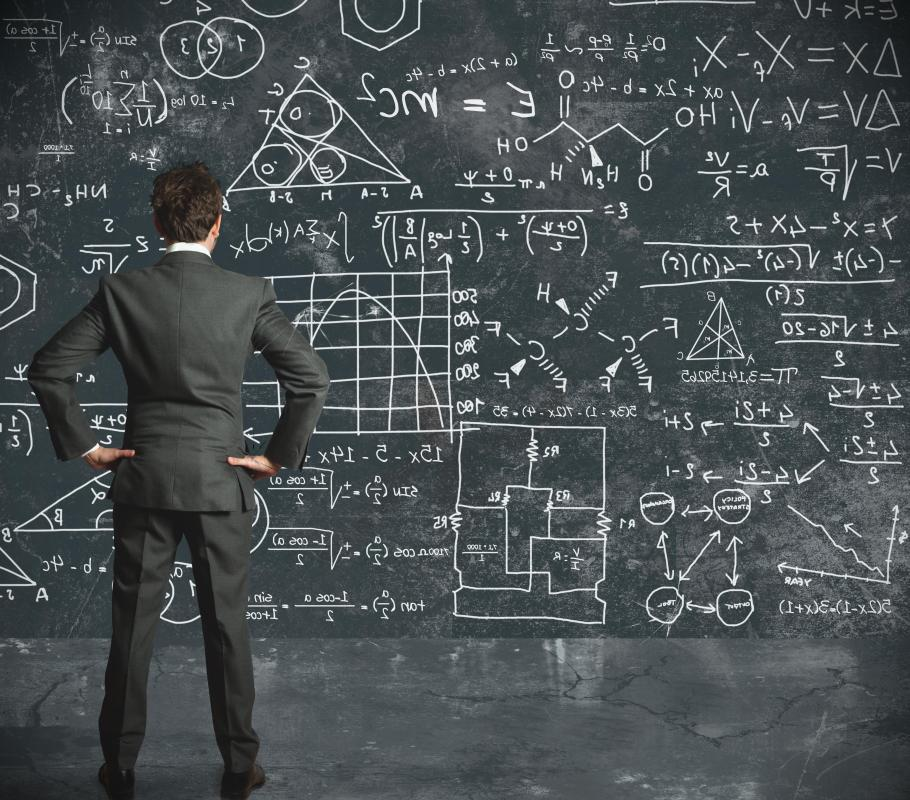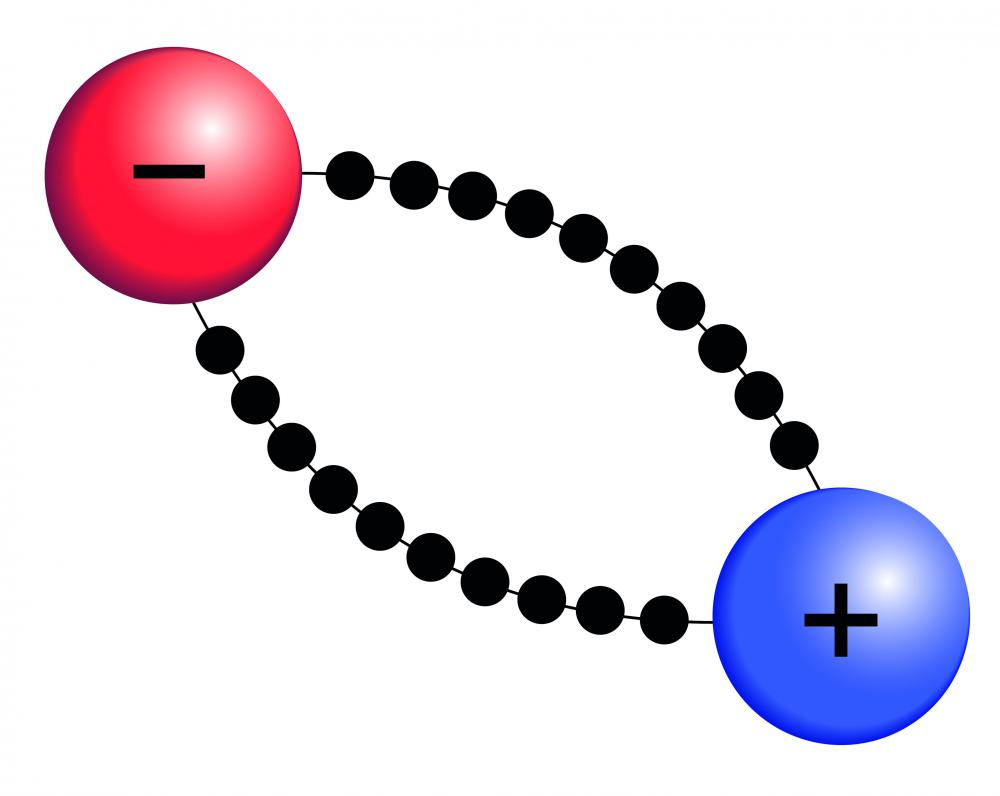# What Is Coulomb's Law?

Alex Paul
Alex PaulCoulomb’s law in physics describes the interaction of two charged particles.

Coulomb’s law in physics describes the interaction of two charged particles. It states that the force between the particles depends on the size of the individual charges and the square of the distance between them. There are two forms of the law, known as the scalar and vector forms, depending on whether just the magnitude of the force or both the magnitude and the direction of the force are required.A coulomb is a unit of electrical charge and is defined as the charge that flows with a constant current of one ampere during one second.

Coulomb’s law is one of the most famous equations in electrostatics, which is the study of how electrically charged particles interact with one another. It was originally discovered by Charles Augustin de Coulomb, a French scientist, in 1783. Without this discovery, scientific understanding of electric and magnetic fields would have been much more difficult.

The basic form of Coulomb’s law, in words, states that the force between two particles with an electric charge is proportional to the multiplication of the two charges divided by the square of the distance. This means particles with a larger charge exert a larger force on one another than those with a weaker charge. It’s important to note that, to find the absolute value of the force between the particles, a constant known as “Coulomb’s constant” is required.

In Coulomb’s law, the force is inversely proportional to the distance squared rather than just the distance itself. If a quantity is inversely proportional to another, then it means the size of one decreases as the other increases. This means that, if the distance between two particles doubles, the force between them is four times smaller rather than just two times. The reason is that the electric field from one particle spreads out in a sphere shape, which means the further away from the particle, the more diluted the force.

Another important factor to consider in Coulomb’s law is that if it is used in its vector form, which means that both size of the force and direction are included, it can be used with both positive and negative particles. The result is that two charges of the same type repel each other, while those of the opposite type attract. Physicists usually use the vector form of Coulomb’s law in calculations, because it provides more information about the force on each particle.

A useful aspect of Coulomb’s law is that it can be easily applied to more than two particles. This is known as the law of superposition, which states that the total force on any one particle is the sum of the individual forces. To add forces in this way requires the vector form of Coulomb’s law.

## You might also Like## Discuss this Article

Forgot password?
###### Register:
•Coulomb’s law in physics describes the interaction of two charged particles.
•A coulomb is a unit of electrical charge and is defined as the charge that flows with a constant current of one ampere during one second.